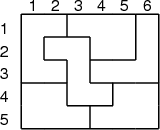# Internet Problem Solving Contest

## Problem R – Reverse quick search

After creating problem Q, we thought it would be entertaining to put you into problemsetters’ shoes for a moment. In this task you will find out a way to create test data for problem Q.

Problem specification

You are given integers R, C, and A such that A divides RC.

Your task is to take a R × C rectangle and divide it into polygons. Each of the polygons must consist of exactly A unit squares. All polygons must be distinct, except for two polygons that must be identical.

Note: We call two polygons identical iff one of them can be obtained from the other by a combination of translations and rotations. Note that it is not allowed to use axis symmetry – i.e., if a polygon is just a mirror image of another one, they are considered distinct for the purpose of this problem.

Input specification

The input contains a single line with the three integers R, C, and A.

Output specification

After you divide the rectangle into N = RC∕A polygons, number them from 1 to N (in any order you wish).

Output R rows with C integers in each of them. The c-th integer in the r-th row must be the number of the polygon that contains the cell (r,c).

Any valid output will be accepted.

Example

 input 5 6 5
 output 1 1 2 2 2 3  1 4 4 2 2 3  1 1 4 3 3 3  6 6 4 4 5 5  6 6 6 5 5 5